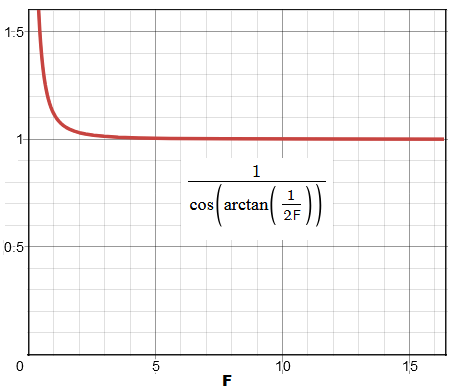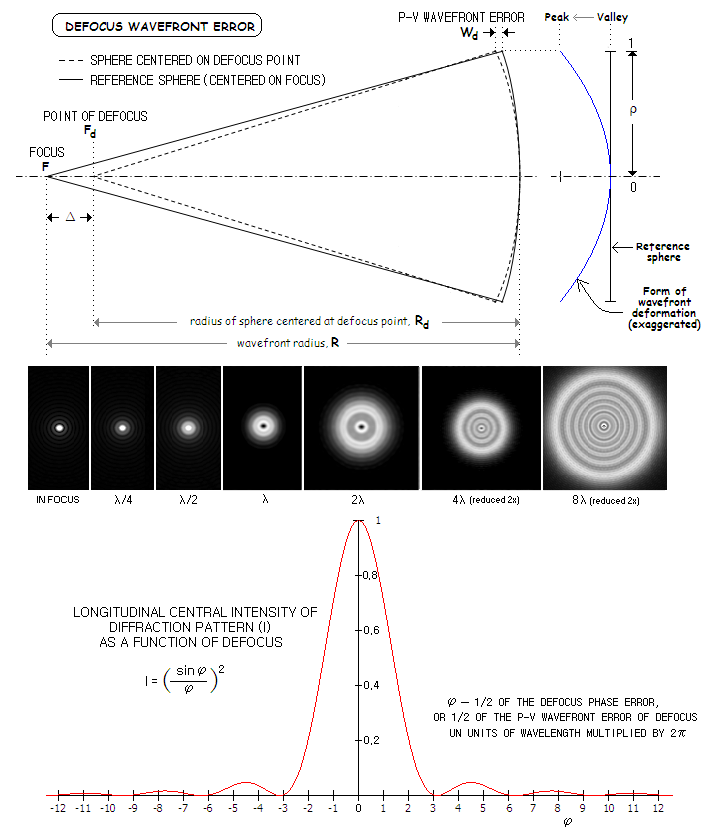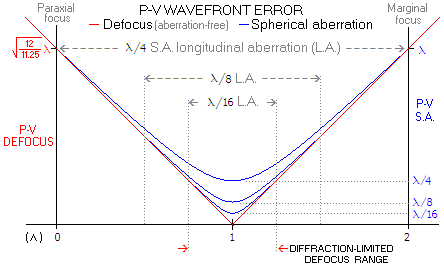telescopeѲptics.net          ▪▪▪▪                                             CONTENTS

# 4.4. DEFOCUS ABERRATION

Formally, defocus wavefront aberration results from the image formed by a telescope objective being observed not at the location of the Gaussian image point, but at a point longitudinally displaced from it. In more practical sense, defocus error occurs when point of observation is displaced from best, or diffraction focus, a point with peak diffraction intensity embedded into longitudinal extension of the central maxima. Longitudinal shift away from this point, to either side, results in gradual decrease of central intensity, with the energy spreading from the central maxima out to the rings area.

In its pure form, defocus occurs in aberration-free aperture, in which case the longitudinal defocus error equals longitudinal displacement from Gaussian image point, and the corresponding P-V wavefront error is given by the sagitta difference between two reference spheres in the entrance pupil: one centered at Gaussian focus (F on the illustration below), and the other at the point of observation (Fd). Hence, longitudinal defocus is given by the difference between the two radii, Ld=R-Rd, where R is the pupil-to-image distance (equaling focal length for object at infinity) and Rd the distance from pupil to the point of defocus.

The corresponding P-V wavefront error equals the differential between two sphere's sagitta, given with the expansion series Wd=[(1/Rd)-(1/R)]D2/8 + [(1/Rd3)-(1/R3)]D4/32 + ... = ΔD2/8RRd + Δ(R3+Rd3)D4/32R3Rd3 + ..., where Δ is the longitudinal defocus (thus Rd=R+Δ). Since the second term already is smaller than the first term by a factor ~3ΔD2/4R2~3Δ/4F2, a full aperture P-V defocus wavefront error, for Δ values of ~λ (wavelength unit) and smaller is accurately (within a small fraction of percentage point) given by:

Wd = [(1/Rd)-(1/R)]D2/8 =  ΔD2/8RRd =  Δd2/2RRd      (23)

with D being the aperture diameter. The error sign is positive when the OPD from the aberrated to focal point is larger than that from the point at identical zonal height at the reference sphere, and negative for smaller OPD (on the illustration bellow, the wavefront error is numerically negative).Note that this simplified expression assumes the cosine factor of the marginal ray path to be 1, i.e. ray path parallel with the optical axis; the actual P-V error is larger by a factor of 1/cos[arctan(1/2F)], with the difference reaching 1% in an f/3.5 system (it is also not the actual value, since the two ray paths toward focus point have slightly different angles, but the difference is entirely negligible). As a graph at left shows, the difference is practically negligible for nearly all actual systems; even at f/1, when the difference between the simpler and more exact form for the P-V error exceeds 11%, the actual Strehl value for the "diffraction limited" level (0.80) given by the former would be 0.76.

Figure bellow shows the diagram of defocus error (top), as well as the changes in the appearance of otherwise aberration-free diffraction pattern for unobstructed aperture caused by increasing defocus error (bottom) and change in the longitudinal peak intensity as a result of defocus .FIGURE 47: TOP: P-V wavefront error of defocus is determined by the difference in the radii (Δ) between the wavefront sphere and an arbitrary sphere with the same vertex, centered at axial point Fd separated from the focus point F (left). The corresponding form of wavefront deviation from the sphere centered at Fd is a paraboloid, either concave or convex, depending on the direction of axial shift (right). MIDDLE: Diffraction images for selected P-V wavefront errors of defocus. BOTTOM: Change in the longitudinal central intensity (Strehl) of the defocused pattern, for otherwise aberration-free aperture. With φ=2πWd and Wd=φ/2π, the first intensity zero at φ/2=π corresponds to Wd=1; successive minimas come at φ/2=π, 2π, 3π... and the corresponding P-V wavefront errors are 1, 2, 3... Knowing that defocus P-V wavefront error in units of wavelength is given by
/8F2, with being the linear defocus, the corresponding linear equivalent for the minimas are 8λF2, 16λF2, 24λF2...

In such instances when this is not the case - such as the presence of spherical aberration, or astigmatism - certain amount of defocus from the Gaussian image point is necessary to shift to the actual best focus location. In other words, defocus can be balanced with another aberration in order to have the combined aberration minimized (for instance, best focus in the presence of spherical aberration is at the point halfway between paraxial and marginal focus, thus combines spherical aberration and defocus).

In everyday's jargon, "defocus" has somewhat different meaning: it is simply an axial deviation from best focus location, correctable by mere refocusing.

Back to best focus being coinciding with the Gaussian, defocus P-V wavefront error is measured as the optical path difference between a perfect reference sphere for the point other than the Gaussian, and a perfect reference sphere for Gaussian image point. It is expressed as:

Wd = Pρ2             (24)

with ρ being the in-pupil ray height in units of pupil radius, and P the peak aberration coefficient for defocus (equal to the P-V wavefront error) given by Eq. 23. It changes with the square of pupil height.

For very small relative difference between the two reference radii, such as those for typical defocus values in telescopes, Eq. 24 can be written as

Wd ~ (Rd-R)D2/8R2 ~ ΔD2/8R2            (24.1).

with Δ being the longitudinal defocus (effectively, the difference in radii between the two reference spheres).

Since this approximation differs from the exact value by a factor of Rd/R, it is as good as the exact value for all practical purposes (for instance, the relative difference between the true value and approximation for 1mm defocus in a 200mm f/10 system is 0.9995, and still only 0.9975 in a 80mm f/5 system).

With R equaling the system focal length f for object at infinity, (D/R)2=1/F2, where F is the system focal ratio f/D, and the peak aberration coefficient for defocus, equaling the P-V wavefront error, is:

P = -Δ/8F2,           (24.2)

It is radially symmetrical aberration, with the geometric blur diameter given simply by Bd=Δ/F. Substituting for Δ from Eq. 24.2, geometric defocus blur diameter in terms of the P-V wavefront error is Bd=8P, in units of λF (which implies P=0.305λ for the blur equal to Airy disc diameter). In units of the Airy disc diameter (2.44λF) for defocus is:

Bd = 8P/2.44λ  = -Δ/2.44λF2          (25)

or Bd=745Δ/F2 for 550nm (0.00055mm) wavelength. Since the peak aberration coefficient P equals the P-V wavefront error Wd for ρ=1, the blur size in Airy disc diameters is, from Eq. 26, also given by Bd=8W'd/2.44, with W'd being the P-V wavefront error of defocus in units of the wavelength. Actual diffraction pattern, when defocused to a given W'd value (from about 0.5 up) is somewhat larger than indicated by Bd, due to the actual pattern always having certain depth, hence showing a portion of wider external energy spread away from the focal point.

The peak defocus aberration coefficient - i.e. P-V wavefront error - and the RMS wavefront error relate as ωd=P/√12=Wd/12. This implies that the P-V wavefront error of defocus corresponding to "diffraction-limited" level, (i.e. to ω=1/180, for 0.80 Strehl) is P=12/180=1/15=0.258, in units of wavelength.

The RMS wavefront error relates to blur diameter Bd as ω=2.44Bd/√768. The RMS blur spot radius for defocus, rRMS=PF8. The RMS spot diameter expressed in Airy disc diameters, is RRMS=Wd8/1.22 in terms of the P-V wavefront error, and RRMS=46ωd/1.22in terms of defocus RMS error ωd.

In terms of phase error, the peak value of defocus phase aberration is given as Φd=πΔ/4λF2, in units of the full phase of 2π radians (thus giving the P-V wavefront error in units of wavelength when divided by 2π). The corresponding Strehl ratio is given by S=sinc2(Φd/2)=[sin(Φd/2)/(Φd/2)]2=[sin(180Δ/8λF2)/(πΔ/8λF2)]2.

EXAMPLE
: Thus, an f/10 system defocused by Δ=0.1mm has the P-V wavefront error of defocus given by Wd=P=0.1/800=0.000125 or 1/4.4 wave, with the corresponding geometric blur diameter of Bd=74.5/100=0.745 Airy disc diameters, both for 550nm wavelength. This same linear defocus in an f/5 system will produce Wd=P=0.1/200=0.0005 or 1/1.1 wave P-V wavefront error, with the corresponding geometric blur diameter of Bd=74.5/25=3 Airy disc diameters.

The peak defocus phase aberration is Φd=1.43 radians, or 1/4.4 of the full phase of 2π.  The corresponding Strehl is S=0.84.

An important property of a telescope is its defocus sensitivity. As Eq. 24.2 implies, it is proportional to the longitudinal defocus Δ, and inversely proportional to F2. Since P equals the P-V wavefront error Wd of defocus, expressing it in units of the wavelength gives W/λ=-Δ/8λF2. Hence taking the maximum allowable P-V wavefront error of defocus Wd/λ, in either direction from the exact focus, the corresponding longitudinal range (including both sides of defocus) within which defocus error will not exceed this level is given by:

Ld = 16WλF2          (26)

where W=Wd/λ is the defocus P-V wavefront error in units of the wavelength. In terms of the RMS wavefront error ω, Ld=323ωλF2.

It is sometimes called focus depth, not to be confused with the meaning of the same term in photography and, sometimes, ophthalmology. The latter has little to do with the actual optical quality, merely indicating the range of geometric defocus within which a circle of confusion (defined as vertical cross-section of an assumed perfect converging/diverging cone) does not exceed certain size, usually 0.2mm or 0.01 inch, when magnified to the usual  8x10 format, observed from the usual ~1 foot distance. That amounts to the angular blur size of little over 2 arc minutes, at best referring to the defocus magnitude in an imaginary perfect system.

Focus depth in telescopes is also not to be confused with field depth, a photographic term referring to the range of distance in object space within which object image retains needed quality level; given, with respect to infinity focus, by Δ=± 8Wn (o-f)2 /[D2-8(o-f )Wn], where o is the focused distance, larger than f for close objects, f and D the system focal length and aperture diameter, respectively, and Wn the nominal P-V wavefront error of defocus, it is irrelevant in astronomy, where all objects are very distant.

Taking W=0.258 for 1/3.87 wave of defocus (0.0745 wave RMS) allowable for the conventional "diffraction-limited" level of 0.80 Strehl, gives the corresponding ± range of defocus as Ld=4.13λF2. For an f/10 system and λ=0.00055mm, it gives 0.227mm.

This is, of course, an idealized system. In the presence of spherical aberration - rather common scenario - allowed defocus is significantly smaller. For a system with the P-V wavefront error W of lower-order spherical aberration in units of the wavelength - assuming W<0.25 for better than "diffraction-limited" level - with the corresponding longitudinal aberration LAs=64λWF2, allowed ± defocus range for remaining at the conventional "diffraction-limited" level, or better, is given by Lds=xLAs, with x=(1-16W2)0.5/415W obtained from Eq. 6. Hence diffraction-limited defocus range in the presence of spherical aberration is given by:

Lds = 16λ(1-16W2)0.5F2/15             (26.1)

Taking W=1/6, for λ/6 wave P-V of spherical aberration, and λ=0.00055mm, allowable defocus range in an f/10 system (so F=10) is 0.169mm (note that "defocus range" in this context doesn't equal defocus error; at the best focus location, lower-order spherical aberration is already combined with longitudinal defocus equaling one half of the longitudinal spherical aberration).FIGURE 48: Comparative plots for P-V wavefront error of defocus (red) and selected levels of primary spherical aberration (blue). Unlike defocus error alone (in a system with zero spherical aberration), which has linear rate of change from or toward the minimum at the best focus location (1 at the scale representing longitudinal spherical aberration normalized to 2, i.e. 0<Λ<2), spherical aberration causes the rate of change to be slower around best focus location, while tending to equalize with the defocus-alone-error farther away from it. Diffraction limited defocus range for zero-spherical aberration is constant, identical to the longitudinal spherical aberration range for λ/16 wave P-V. In the presence of spherical aberration greater than λ/16 wave, diffraction limited defocus range shrinks: subtly at λ/8 wave, but quickly dropping to zero at λ/4 wave.

For W=0.25, i.e. λ/4 wave of lower-order spherical aberration in the system, allowed defocus to remain within "diffraction-limited" range is zero. Eq. 26.1 is accurate for 0.25≥W≥0.0625; for W=1/16, the diffraction limited defocus range becomes identical to that of aberration-free aperture for ±λ/4 wave of defocus, as shown on the illustration to the left, depicting error function over the range of longitudinal spherical aberration, given with Eq. 6 (units on the horizontal axis are Λ, longitudinal aberration normalized to 2 for λ/4 P-V error of spherical aberration; note that the P-V wavefront error of defocus is slightly larger for given RMS error). For W<1/16, Eq. 26.1 gives slightly larger values for diffraction-limited defocus range, than what is obtained for the λ/4 wavefront error of defocus range. This is a consequence of the defocus RMS error being, for given P-V wavefront error, slightly smaller - by a factor of (11.25/12)0.5 - than the RMS error of spherical aberration. For zero wavefront error of spherical aberration (W=0), Eq. 26.1 gives diffraction-limited range larger by a factor of (12/11.25)0.5 than the range for λ/4 wave of defocus. That is the true diffraction limited defocus range, for the RMS error producing 0.80 Strehl at either end; the corresponding P-V wavefront error of defocus is, as mentioned before, 0.258λ.

In aberration-free systems with central obstruction, defocus RMS error is smaller by a factor of (1-o2) than in unobstructed aberration-free system, o being the relative c. obstruction size in units of the aperture diameter. Consequently, the Strehl ratio in terms of peak defocus phase aberration Φd, for linear defocus Δ, is given by

S=sinc2(Φd(1-o2)/2)={sin[Φd(1-o2)/2]/[Φd(1-o2)/2]}2=

={sin[(180Δ/8λF2)(1-o2)]/(πΔ/8λF2)(1-o2)]2.

If spherical aberration is present, the RMS wavefront error of defocus is reduced by a factor of (1-o2)2, and its defocus error changes similarly to that in clear aperture with spherical aberration. Hence, in terms of error added by defocusing, obstructed system with spherical aberration has wider defocus range than unobstructed system with identical level of spherical aberration. However, its starting point - best focus quality level - is degraded by the effect of obstruction. In order to have any diffraction-limited defocus range, an obstructed aperture needs to have normalized peak diffraction intensity greater than 0.80. This implies the optics Strehl better than 0.8/(1-o2)2. With even moderate linear obstruction ratio o=0.25 (i.e. 0.25D), a system needs to have the optics Strehl better than 0.91 (i.e. better than 1/6.1 wave P-V of spherical aberration level). A 0.95 optics Strehl system, or slightly better than 1/8 wave of lower order spherical level, cannot add more than about 1/24 wave of P-V wavefront error and remain diffraction limited. Unobstructed system with identical correction error can add three times as much of P-V wavefront error (1/8 wave), implicating roughly three times wider diffraction-limited defocus range for given relative aperture.